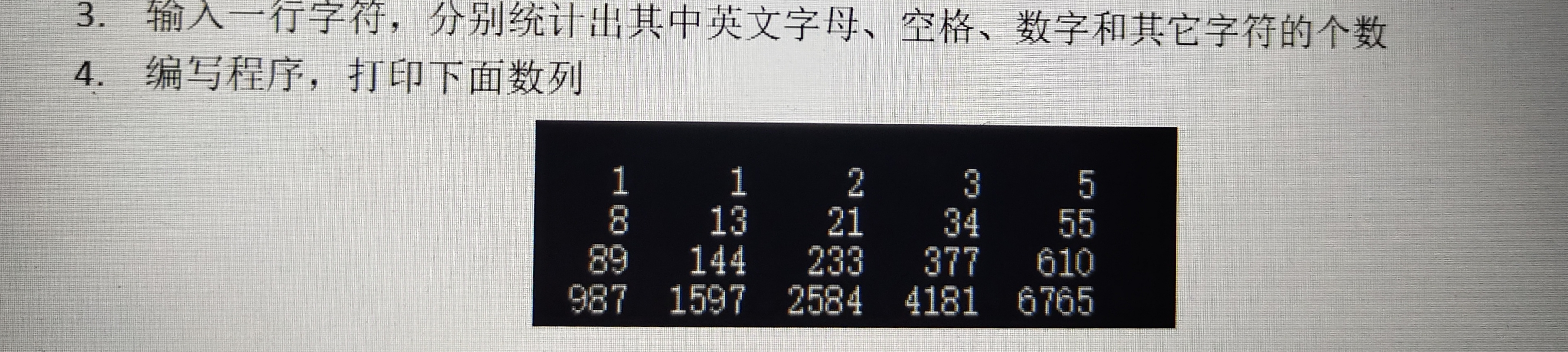2021-06-20 13:47

# 大佬们，帮帮忙，2道c语言题• 写回答
• 关注问题
• 收藏
• 邀请回答

#### 1条回答默认 最新

•像向日葵一样~ 2021-06-20 14:58
已采纳

（1）

``````#include <stdio.h>

int main()

{
char c;

int letters=0,space=0,digit=0,other=0;

printf("请输入一行字符：");

while ((c=getchar())!='\n')

{
if (c >= 'a'&&c <= 'z' || c >= 'A'&&c <= 'Z')

{
letters++;

}

else if (c == ' ')

{
space++;

}

else if (c >= '0'&&c <= '9')

{
digit++;

}

else

{
other++;

}

}

printf("字母数:%d\n空格数:%d\n数字数:%d\n其他字符:%d\n",letters,space,digit,other);

return 0;

}``````

（2）

该题就是用c语言求斐波那契数列的前20项

``````#include <stdio.h>
int f(int n)       // 求斐波那契数列的第n项
{
if(n==1||n==2)
return 1;
else
return f(n-1)+f(n-2);
}
void main()
{
int n;
for(n=1;n<=20;n++)
{
printf("%8d",f(n));     // 要求每个元素占8位
if(n%5==0)
printf("\n");
}
}
``````
已采纳该答案
打赏 评论